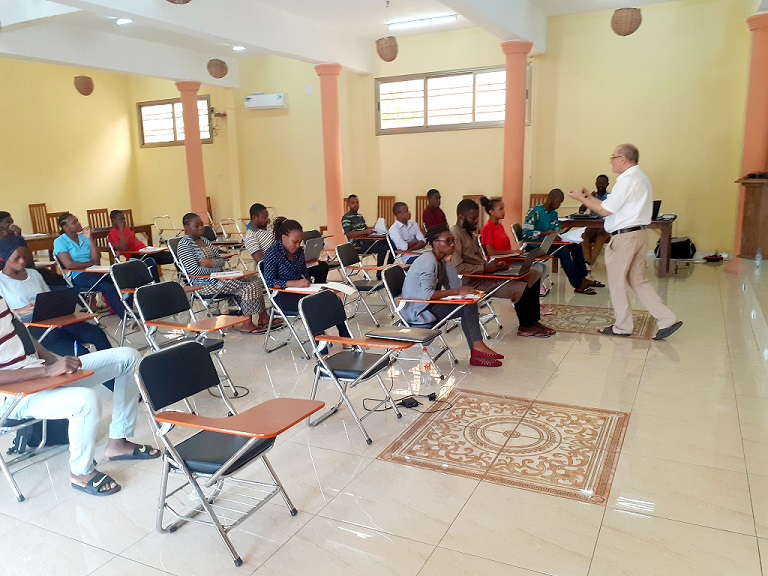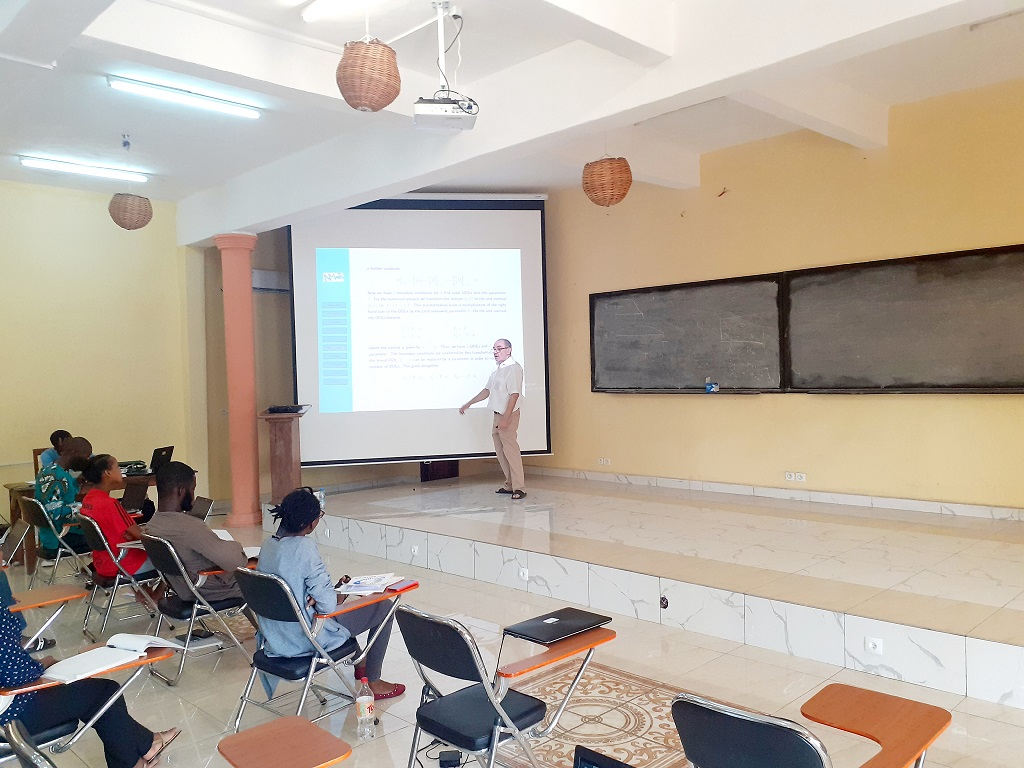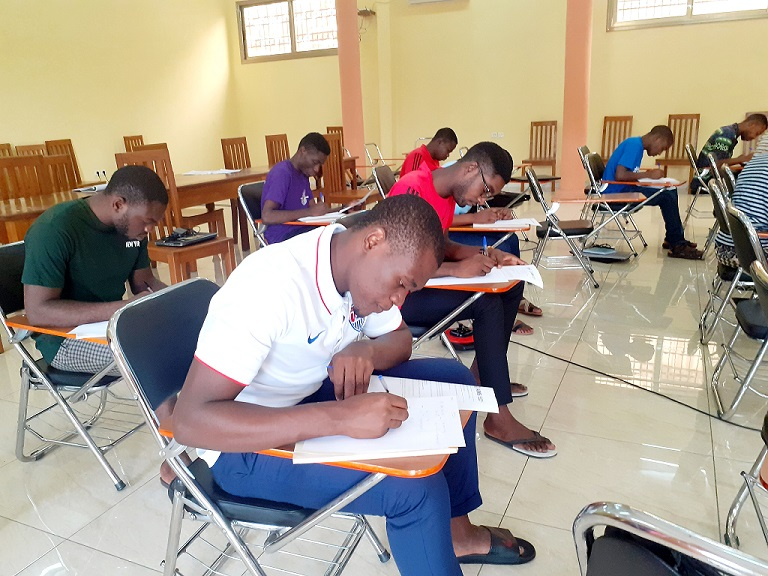April 14, 2021

# Prof. GEORG BADER Introduces Students To Scientific Computing With Python

AIMS Cameroon’s visiting lecturer Prof. Georg Bader is on campus for a 30-hour lecture season on a variety of Mathematics courses. At a pace of two hours a day, Prof. Bader is shaping the students’ mastery of Scientific Computing and Numerical Analysis.

On April 14th, Prof. Bader introduced Structured Master’s Degree students to the depths of Scientific Computing with Python. Python is a general-purpose programming language which can be used for a wide range of computational tasks as well as data mining and software development.

Defining Scientific Computing as a collection of tools, techniques, and theories required to solve on a computer mathematical models of problems in Science and Engineering, Prof. Bader explained that this set of mathematical theories and techniques is called Numerical Analysis and constitutes a major part of Scientific Computing.

He said the development of the electronic computer signaled a new era in the approach to the solution of scientific problems. “Many of the numerical methods that had been developed for the purpose of hand calculation had to be revised and sometimes abandoned. Considerations that were irrelevant or unimportant for hand calculation now became of utmost importance for the efficient and correct use of a large Computer System. Many of these considerations – programming languages, operating systems, management of large quantities of data, correctness of programs – were subsumed under the new discipline of Computer Science, on which Scientific Computing now depends heavily,” explained Prof. Bader in his introduction.Focus was on the simulation of Ordinary and Partial Differential Equations.

But mathematics itself, he added, continues to play a dominating role in scientific computing because it provides the language of the mathematical models that are to be solved and information about the suitability of a model provides the theoretical foundation for the Numerical methods and, increasingly, for many of the tools from Computer Science.

Due to the diversity of this subject, Prof. Bader and his students this morning mainly focused on the simulation of Ordinary and Partial Differential Equations.

At AIMS Cameroon, the course is compulsory for the ten students in Fundamental Science but some Climate Science and Data Science students are also taking it.

Under the supervision of Prof. Bader and Lecture Assistant James Berinyuy Njong Jr., the students took a quiz. “AIMS runs a continuous evaluation for each course through quick quizzes, weekly assignments and a final group assignment wherein the students team up to work on mini projects where they leverage the tools from the course they are completing to solve practical problems,” explained James Berinyuy Njong Jr.The students also took a quiz as part of their continuous evaluation

Prof Georg is a retired Professor for Scientific Computing and Numerical Analysis at Brandenburg University of Cottbus-Senftenberg, Germany. He completed his PhD in Applied Mathematics at the University of Heidelberg. He is a frequent lecturer at the Ghana and Cameroon centres of the African Institute of Mathematical Science. His areas of expertise are numerical analysis, discretization of ordinary and partial differential equations, parallel algorithms and large-scale simulations.

Prof. Bader’s main interests of Research are: the Analysis and Development of Discretization’s for Ordinary and Partial Differential Equations, Efficient Implementation and Parallelization of Numerical Methods for Differential Equations■